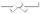# Search Results

Searching for: Visualizable

## Individual Exercises

0 / 10

Consider the following class definition:
Object data;
}
Create a new node with info 'D' and insert it at the end...0 / 10

Consider the following class definition:
Object data;
}
Write a while loop to make q refer successively to each...0 / 10

Consider the following class definition:

``class Link{ public Object data; public Link next; } ``

In the method below, you will receive a...0 / 10

Consider the following class definition:
Object data;
}
Return a reference to the second to last node (null if...0 / 10

Consider the following class definition:
Object data;
}
List L has a reference p to the first node. Write code...0 / 10

Consider the following class definition:
Object data;
}
List L has a reference p to the first node. Write code...0 / 10

Consider the following class definition:
Object data;
}
List L has a reference p to the first node. Write code...0 / 10

Consider the following class definition:
Object data;
}
List L has a reference p to the first node. Write code...0 / 10

Consider the following class definition:
Object data;
}
p is a reference to the first node in a List L. Create a...0 / 10

Consider the following class definition:
Object data;
}
List L has a reference to the first node. Write code that...0 / 10

Consider the following class definition:
Object data;
}
p is a reference to the first node in a List L. Integer...0 / 10

Consider the following class definition:
Object data;
}
p is a reference to the first node in list L. Integer val...0 / 10

Consider the following class definition:
Object data;
}
p is a reference to the first node of list L. Integer ref...0 / 10

Consider the following class definition:
Object data;
}
p is a reference to the first node in a list L. Return a...0 / 10

Consider the following class definition:
Object data;
}
List L has a reference to the first node. Given a...0 / 10

Consider the following class definition:
Object data;
}
p is a reference to the first node of List L. Link q is a...0 / 10

Consider the following class definition:
Object data;
}
p is a reference to the first node of List L. q is a...0 / 10

Consider the following class definition:
Object data;
}
Use four assignment statements, each referring to variable...0 / 10

Consider the following class definition:
Object data;
}
Create a new node with info 'A' and insert it at the...0 / 10

Consider the following class definitions:

``public class LinkedChain<T> { private Node<T> firstNode; private int...``0 / 10

Consider the following class definitions:

``public class LinkedChain<T> { private Node<T> firstNode; private int...``0 / 10

Consider the following class definitions:

``public class LinkedChain<T> { private Node<T> firstNode; private int...``0 / 10

Consider the following class definitions:

``public class LinkedChain<T> { private Node<T> firstNode; private int...``0 / 10

Consider the following class definitions:

``public static final class LinkedChain<T> { private Node<T> topNode; private int...``0 / 10

Consider the following class definitions:

``public static final class LinkedChain<T> { private Node<T> topNode; private int...``